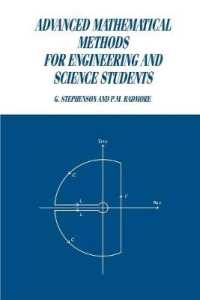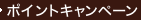•### Advanced Mathematical Methods for Engineering and Science Students

• 提携先の海外書籍取次会社に在庫がございます。通常2週間で発送いたします。
重要ご説明事項
1. 納期遅延や、ご入手不能となる場合が若干ございます。
2. 複数冊ご注文の場合、分割発送となる場合がございます。
3. 美品のご指定は承りかねます。
• ≪洋書のご注文につきまして≫ 「海外取次在庫あり」および「国内仕入れ先からお取り寄せいたします」表示の商品でも、納期の目安期間内にお届けできないことがございます。あらかじめご了承ください。

• 製本 Paperback:紙装版/ペーパーバック版／ページ数 255 p.
• 言語 ENG
• 商品コード 9780521368605
• DDC分類 510

### Full Description

This book provides a solid foundation to a number of important topics in mathematics of interest to science and engineering students. The authors' approach is simple and direct, the emphasis being on the analytical structure and applications of the material. The text is virtually self-contained, assuming only that the student has received a good basic course in ancillary mathematics. Each chapter contains a large number of worked examples, and concludes with problems for solution, with answers given in the back of the book. There is no comparable text that covers this material in such a concise form. This book will be of great value to undergraduates in physics, chemistry, theoretical biology, and in all engineering disciplines, as a source book of advanced mathematical methods, and also to postgraduate students as a revision text.

### Table of Contents

`1. Suffix notation and tensor algebra2. Special functions3. Non-linear ordinary differential equations4. Approximate solutions of ordinarydifferential equations5. Contour integration6. Applications of contour integration7. Laplace and Fourier transforms8. Partial differential equations9. Calculus of variations.`